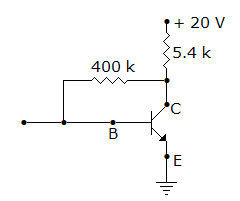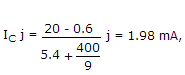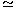# Electronics and Communication Engineering - Analog Electronics - Discussion

12.

In figure, VEB = 0.6 V, β = 99. Then VC and IC are[A]. 9.3 V and 1.98 mA respectively [B]. 4.6 V and 1.98 mA respectively [C]. 9.3 V and 0.02 mA respectively [D]. 4.6 V and 0.02 mA respectively

Explanation:VC = 20 - 1.98 x 10-3 x 5.4 x 1039.3 V.

 Sandeep said: (Jan 26, 2015) Why 400/9 is taken?

 Arif said: (Feb 28, 2015) Use these formulas: Ib = (Vcc-Vbe)/(Rb+BRc). Ic = BIb. Vc = Vcc-IcRc.

 Sivaiah said: (Jul 28, 2016) In question, they mention it as Veb but it is Vbe.

 Chenelyn Cedron said: (Aug 3, 2016) @Sandeep It's 400/99, just a typo error. In fact, if you are bookish enough and you hate approximations, your first equation might look like this: Vcc = V{5.4k} + V{400k} + V{be}. Vcc = ((B)(Ib) + 1)(5.4k)(Ic)/B + (400k)(Ic)/B + V{be}. Substituting the given gives: 20 = (99 + 1)(5.4k)(Ic)/(99) + (400k)(Ic)/(99) + 0.6. This would give you an Ic of 2.043 mA. Then since Vce = Vc = Vcc - IcRc, Vc = 20 - (2.043mA)(5.4k) = 8.97 V. Pretty close to letter A.

 Neeraj said: (Mar 21, 2017) Operating Vbe should be 0.7.

 Prutha said: (Oct 19, 2017) I am Not clear with the solution. Please explain.

 Aman said: (Jan 10, 2019) Apply kvl from 20-Ic * 5.4-Ib * 400-0.6 = 0. You will get Ib=. 02ma.then Ic=BIb=1.98ma. Now you can easily find Vc.

 Karthik said: (Jan 28, 2019) What is 400/9?

 Vasanthakumara D said: (Oct 6, 2019) This question 400/9 how come 9?

 Rajani said: (Oct 10, 2019) It should be 400/99 not 400/9.